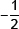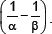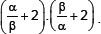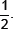# Polynomials-Sample Papers

Class X Math
Test For Polynomials
Max Marks : 50
Max Time : 1 hour 10 Mins
1.  What is the value of p(x) = x2 – 3x – 4 at x = –1?            (3 marks)
2.  What is the zero of 2x + 3?            (2 marks)
3.  The Coefficient of x and the constant term in a linear polynomial are 5 and –3 respectively. Find its zero.            (2 marks)
4.  Find the product of zeroes of the quadratic polynomial 3x2 + 5x – 2.            (2 marks)
5.  If a fifth degree polynomial is divided by a quadratic polynomial, write the possible degree of the quotient.            (3 marks)
6.  For what value of k, (–4) is a zero of the polynomial x2 – x – (2k + 2)?            (3 marks)
7.  The sum and product of the zeroes of a quadratic polynomial areand –3 respectively. What is the quadratic polynomial?            (2 marks)
8.  If 1 is a zero of the polynomial p(x) = ax2 – 3 (a – 1) x – 1, then find the value of a.            (3 marks)
9.  Write a quadratic polynomial, the sum and product of whose zeroes are 3 and –2 respectively.            (2 marks)
10.  If α and β are the zeroes of a quadratic polynomial x2 + x – 2 then find the value of(3 marks)
11.  If α and β are the zeroes of x2 + px + q then find the value of(3 marks)
12.  Find the zeroes of 2x2 – 8x + 6.            (3 marks)
13.  Find the value of ‘k’ such that the quadratic polynomial 3x2 + 2kx + x – k – 5 has the sum of zeroes as half of their product.            (3 marks)
14.  Find the cubic polynomial whose zeroes are 5, 3 and –2.            (3 marks)
15.  If 1 is a zero of x3 – 3x2 – x + 3 then find all other zeroes.            (2marks)
16.  Find all the zeroes of 2x4 – 3x3 – 3x2 + 6x – 2, if two of its zeroes are 1 and(2 marks)
17.  Find the zeroes of the quadratic polynomial 4x2 – 6 – 8x and verify the relationship between the zeroes and the coefficients of the polynomial.            (2 marks)
18.  Find the zeroes of the quadratic polynomial 4x2 – 6 – 8x and verify the relationship between the zeroes and the coefficients of the polynomial.            (2 marks)
19.  Find a quadratic polynomial whose zeroes are –12 and 4 and verify the relationship between the zeroes and the coefficients.            (3 marks)
20.  If the polynomial 4x4 + 6x3 + 13x2 + 20x + 7 is divided by another polynomial 3x2 + 4x + 1 then the remainder comes out to be ax + b, find ‘a’ and ‘b’.            (3 marks)